output.to from Sideway
Calculus

Draft for Information Only

Content

Differentiation
Derivative of a function
Differentiation
Derivative
Higher Derivative

Differentiation

Differentiation is concerned with the deriving of the derivative of a function.

Derivative of a function

In many daily life problems, knowing how a dependable variable changed with an independable variable is also very important.

For example a function f :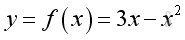Graph of the function is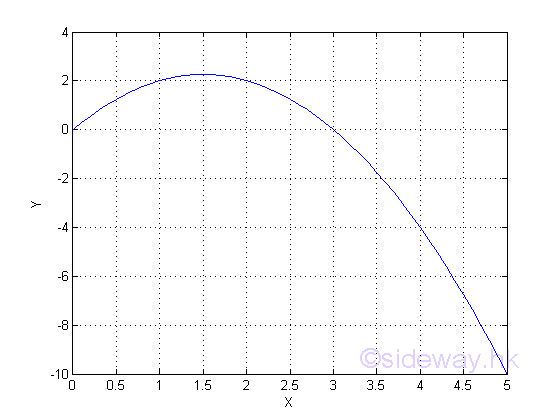When x=1; y=2; and when x=2; y=2 also. And from the graph, the value of y increases then decreases as the value of x increases. The graph can only give a picture of the function and there is no information on how y vary with x

When considering a small change in the independence variable,  there will be a small change in the dependence variable also. Let the small variation of variable x is Δx and the small variation of variable y is Δy. The calculated results of some small changes are:

 x=1 1.1 1.01 1.001 1.0001 x=2 2.1 2.01 2.001 2.0001 Δx=0 0.1 0.01 0.001 0.0001 Δx=0 0.1 0.01 0.001 0.0001 y=2 2.09 2.0099 2.001 2.0001 y=2 1.89 1.9899 1.999 1.9999 Δy=0 0.09 0.0099 0.000999 9.999e-05 Δy=0 -0.11 -0.0101 -0.001001 -0.00010001 Δy/Δx=0/0 0.9 0.99 0.999 0.9999 Δy/Δx=0/0 -1.1 -1.01 -1.001 -1.0001

From the table, the variation of Δy in relation to Δx is obtained. According to the calculated results, the value of Δy varies on both Δx and x. And the reduction of Δx can also reduce the value of Δy.

Therefore Δy/Δx is not a constant but a function of Δx and x. Since Δy/Δx is indeterminate when Δx=0 and the value of Δy is reduced as Δx becomes small, the variation of Δy/Δx as Δx approaching 0  can be evaluated by its limit. This type of limit is named derivative and the notation is: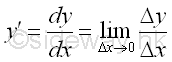Or in the form of function f with number a be the element x of X: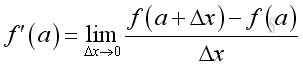And from the table, the derivative of the function f at a, when a=1 and a=2 are as following: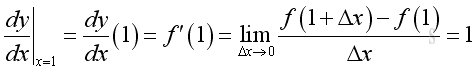and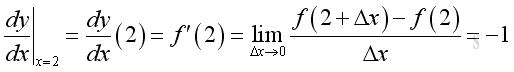Although the value of y is equal to 2 when x=1 and x=2, the variation of Δy in relation to Δx at x=1 is increasing and equal to 1 while the variation of Δy in relation to Δx at x=2 is decreasing and equal to -1.

Differentiation

Instead of using a number a to find the value of the derivative of the function f at a, the Δy/Δx ratio can be expressed in the form of of Δx and x. Imply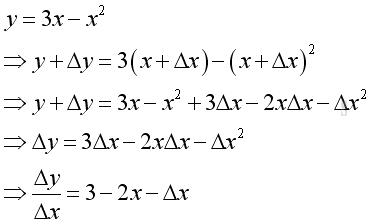And the derivative of the function f  is: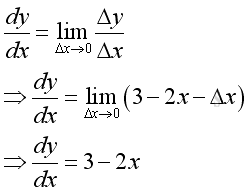Therefore when Δx approaching 0, the derivative of the function  f  is a function of x only. And when x=1, dy/dx=1; and when x=2; dy/dx=-1  as before. From the derivative function, a more clear picture of how Δy in relation to Δx as a function of x is obtained.

Derivative

For a given function f, if the derivative of the function f at c exist, the function f is dfferentiable at c. A function can be differentiated if and only if the function is continuous, in other words, f(c) is defined and f(c) equals to the limit of f(x) when x approaching c. However, not all continuous function are differentiable because the derivative should be defined at c also.

Therefore for a function f that is defined on an open interval (a,b), the function f is dfferentiable on an open interval (a,b) if the function f is dfferentiable at every number c in (a,b).  Imply the derivative f'(c) of a function f at c exist, that is the following limit exists: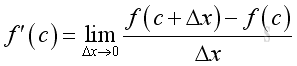And for a function f that is defined on an closed interval [a,b], the function f is dfferentiable on an closed interval [a,b] if the function f is dfferentiable at every number c on the interior (a,b) and is differentiable from one side at its ends.  Imply the derivative f'(c) of a function f at c exist and the following limits exists at the end points of the closed interval [a,b], that is: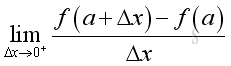and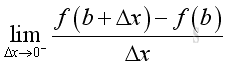The deriviative of a function f is called the first derivative of the function f and is denoted by: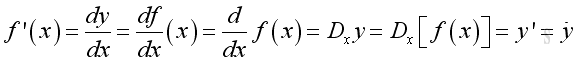The deriviative of the derivative of a function f is called the second derivative of the function f and is denoted by: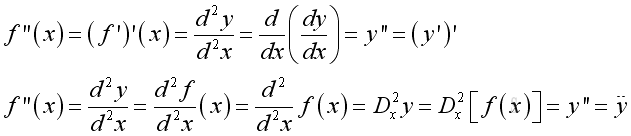And the nth derivative of a function f can also be denoted in the similar way.

Higher Derivative

The deriviative of the derivative of the previous function f can be derived as following. The Δy'/Δx ratio of the first derivative of the function f can be expressed in the form of of Δx and x also. Imply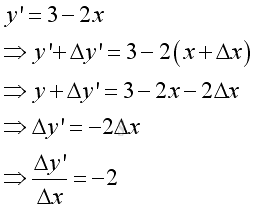And the derivative of the derivative of the function f  is: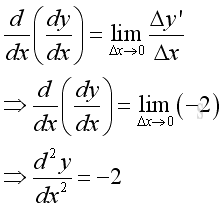From the second derivative function, a very clear picture of how Δy' in relation to Δx is obtained.

Assumed the mentioned function f is the displacement as a function of time. Then the first derivative is the instantaneous velocity as a function of time. The second derivative is the instantaneous acceleration as a function of time.

And higher derivatives can be obtained by repeating the differentiation process , i.e. third derivative by taking the derivative of the second derivative... etcReferences

1. S. James, 1999, Calculus, Brooks/Cole Publishing Co., USA
2. B. Joseph, 1978, University Mathematics: A Textbook for Students of Science & Engineering, Blackie & Son Limited, HongKongID: 110900003 Last Updated: 9/20/2011 Revision: 1 Ref:Home (5)

Management

HBR (3)

Information

Recreation

Hobbies (7)

Culture

Chinese (1097)

English (336)

Reference (66)

Computer

Hardware (149)

Software

Application (187)

Digitization (24)

Numeric (19)

Programming

Web (704)CSS (SC)

ASP.NET (SC)

HTML

Knowledge Base

Common Color (SC)

Html 401 Special (SC)

OS (389)

MS Windows

Windows10 (SC)

.NET Framework (SC)

DeskTop (7)

Knowledge

Mathematics

Formulas (8)

Algebra (20)

Number Theory (206)

Trigonometry (18)

Geometry (18)

Calculus (67)

Complex Analysis (21)

Engineering

Tables (8)

Mechanical

Mechanics (1)

Rigid Bodies

Statics (92)

Dynamics (37)

Fluid (5)

Control

Acoustics (19)

Biology (1)

Geography (1)